# Transfer Function Analysis and Design Tools

This page is a web application that simulate a transfer function.The transfer function is simulated frequency analysis and transient analysis on graphs, showing Bode diagram, Nyquist diagram, Impulse response and Step response.

And use this utility to design the transfer function at a given some poles and zeros or other paramaters.

Transfer Function: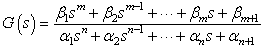Transient analysis condition is nm. maximum n=12

### Example

 G(s)= 10000 s2+50s+10000
The following form is an example to input G(s). Use the right form to simulate.
β=
α=
β=
α=

### Frequency analysis

Bode diagram
Phase  Group delay
Nyquist diagram
Pole, zero
Phase margin
Oscillation analysis

Upper and lower frequency limits:
f1= - f2=[Hz] (frequency limits are optional)

### Transient analysis

Step response
Impulse response
Overshoot
Final value of the step response

Simulation time:
0 - [sec] (optional)

Transfer Function Design Tools ()

The form of α and β values is rewritten by this tool to design the transfer function at given some paramaters.

### Order poles on s-domain

Poles are ordered on s-domain of the transfer function inputted form of α and β. G(s) is rewritten that it solve the following equation.
G(s) = {the transfer function of inputted old α and β}×H(s)

• 1st order system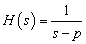p=
• 2nd order system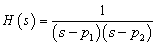p1,p2=±j
p1,p2=exp(±j )

### Order zeros on s-domain

The system of H(s) is setted zeros z, z1 and z2 of a given the following form. The matrix of α and β as transfer function G(s) is rewritten to add zeros. The G(s) is solved the following equation.
G(s) = {the transfer function of inputted old α and β}×H(s)
If α and β was blank, G(s) = H(s).

• 1st order system
H(s)= s - z    z=
• 2nd order system
H(s)=(s - z1 )(s - z2 )
z1,z2= ± j
z1,z2=exp(±j )

### Design from ζ and ω0 on a 2nd order system

Poles are ordered on s-domain of the transfer function inputted form of α and β. G(s) is rewritten that it solve the following equation.
G(s) = {the transfer function of inputted old α and β}×H(s)
If α and β was blank, G(s) = H(s).

2nd order system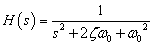•Damping ratio ζ=

### Design the coefficient of s2, s1, s0

G(s) is rewritten that it solve the following equation.
G(s) = {the transfer function of inputted old α and β}×H(s)
If α and β was blank, G(s) = H(s).

the transfer function
H(s)=β1s22s3

β1=0  β1=1  β1=

β2=0  β2=2ζω0 β2=
•Use the above form to input of ω0, ζ

β3=0  β302  β3=
•Use the above form to input of ω0

### Convert frequency→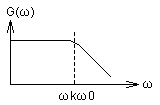ω0(natural angular frequency) from the transfer function of inputted α and β is converted that ω0 is multiplied by a given ωk.

ωk=

### Convert gain

G(s) transfer function of inputted α and β is multiplied by a given K.
G(s)←KG(s)

K=